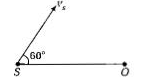A siren emitting a sound of frequency 800 Hz moves away from an observer towards a cliff at a speed of $15{\mathrm{ms}}^{-1}$. Then, the frequency of sound that the observer hears in the echo reflected from the cliff is

(Take, velocity of sound in air=)

(a) 800 Hz

(b) 838 Hz

(c) 885 HZ

(d) 765 Hz

High Yielding Test Series + Question Bank - NEET 2020

Difficulty Level:

An air column, closed at one end and open at the other, resonates with a running fork when the smallest length of the column is 50 cm. The next larger length of the column resonating with the same tunning fork is

(a) 1OO cm (b) 150 cm (c) 200 cm (d) 66.7cm

Concept Questions :-

Standing waves
High Yielding Test Series + Question Bank - NEET 2020

Difficulty Level:

Concept Questions :-

Travelling wave on string
High Yielding Test Series + Question Bank - NEET 2020

Difficulty Level:

A source of sound S emitting waves of frequency 100 Hz and an observer O are located at some distance from each other. The source is moving with a speed of 19.4 ms-1 at an angle of 60° with the source observer line as shown in the figure. The observer is at rest. The apparent frequency observed by the observer (velocity of sound to air 330 ms-1), is(a) 100 Hz

(b) 103Hz

(c) 106 Hz

(d) 97 Hz

Concept Questions :-

Doppler effect
High Yielding Test Series + Question Bank - NEET 2020

Difficulty Level:

A string is stretched between fixed points separated by 75.0 cm. It is observed to have resonant frequencies of 420 Hz and 315 Hz.There are no other resonant frequencies between these two.The lowest resonant frequency for this strings is

(a) 155 Hz

(b) 205  Hz

(c) 10.5 Hz

(d) 105  Hz

Concept Questions :-

Standing waves
High Yielding Test Series + Question Bank - NEET 2020

Difficulty Level:

If n1, n2 and n3 are, are the fundamental frequencies of three segments into which a string is divided, then the original fundamental frequency n of the string is given by

(a) 1/n=1/n1+1/n2+1/n3

(b) 1/√n=1/√n1+1/√n2+1/√n3

(c) √n=√n1+√n2+√n3

(d) n=n1+n2+n3

Concept Questions :-

Travelling wave on string
High Yielding Test Series + Question Bank - NEET 2020

Difficulty Level:

The number of possible natural oscillations of the air column in a pipe closed at one end of length 85 cm whose frequencies lie below 1250 Hz are (velocity of sound 340ms-1) :

(a)4

(b)5

(c)7

(d)6

Concept Questions :-

Wave motion
High Yielding Test Series + Question Bank - NEET 2020

Difficulty Level:

A speeding motorcyclist sees traffic jam ahead of him. He slows down to 36km/h. He finds that traffic has eased and a car moving ahead of him at 18km/h is honking at a frequency of 1392Hz. If the speed of sound is 343m/s, the frequency of the honk as heard by him will be

(a)1332Hz

(b)1372Hz

(c)1412Hz

(d)1454Hz

Concept Questions :-

Doppler effect
High Yielding Test Series + Question Bank - NEET 2020

Difficulty Level:

A wave travelling in the positive x-direction having maximum displacement along y-direction as 1m, wavelength 2π m and frequency of 1/π Hz is represented by

(a) y=sin(x-2t)

(b) y=sin(2πx-2πt)

(c) y=sin(10πx-20πt)

(d) y=sin(2πx+2πt)

Concept Questions :-

Types of waves
High Yielding Test Series + Question Bank - NEET 2020

Difficulty Level:

If we study the vibration of a pipe open at both ends. then the following statements is not true

(a) Open end will be anti-node

(b) Odd harmonics of the fundamental frequency will be generated

(c) All harmonics of the fundamental frequency will be generated

(d) Pressure change will be maximum at both ends

Concept Questions :-

Standing waves